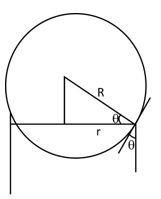Surface tension
Question

# The radius of the bore of a capillary tube is r and the angle of contact of the liquid is $\mathrm{\theta }$ . When the tube is dipped in the liquid, the radius of curvature of the meniscus of liquid rising in the tube is

Moderate
Solution

##$\mathrm{r}/\mathrm{R}=Cos\mathrm{\theta }$$\mathrm{R}=\mathrm{r}/\mathrm{cos\theta }$

Get Instant Solutions